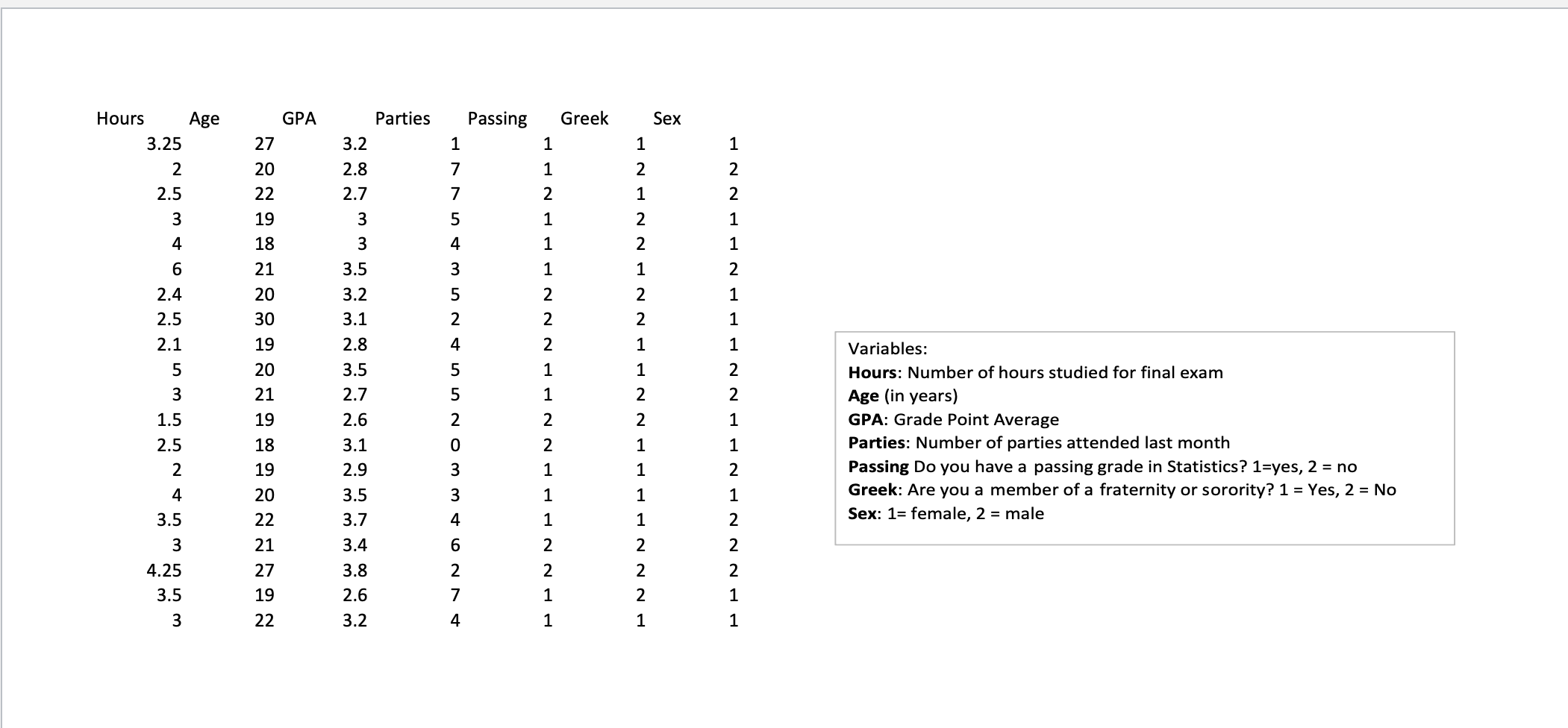# (Solved): Using the data given, answer the following questions. Round to two decimal places.1. What is the av ...Using the data given, answer the following questions. Round to two decimal places.

1. What is the average hours of studying for this sample?

2. What is the average GPA for this sample?

3. What percent of this sample is passing the class?

4. What percent of this sample is female?

5. What is the standard deviation for hours of studying?

Variables: Hours: Number of hours studied for final exam Age (in years) GPA: Grade Point Average Parties: Number of parties attended last month Passing Do you have a passing grade in Statistics? 1=yes, 2 = no Greek: Are you a member of a fraternity or sorority? Yes, No Sex: female, 2 = male

We have an Answer from Expert